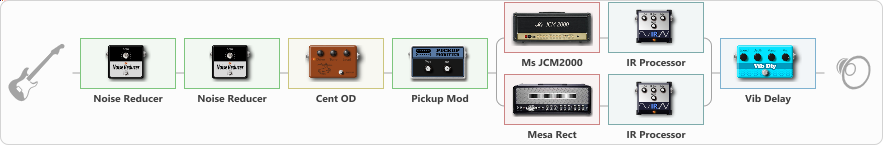# Dual ditst

Discussion in 'ToneLib-GFX presets' started by carlinos3, May 17, 2020.

1. Dual ditst

Preset name: No More Watts

Effects chain:Effect: "Noise Reducer" (Dynamics / Filter), active - "yes"
{
"Sens" = 48
"Mode" = Soft
}

Effect: "Noise Reducer" (Dynamics / Filter), active - "yes"
{
"Sens" = 58
"Mode" = Hard
}

Effect: "Cent OD" (Overdrive / Distortion), active - "yes"
{
"Drive" = 38
"Tone" = 77
"Level" = 60
}

Effect: "Pickup Mod" (Dynamics / Filter), active - "yes"
{
"Mode" = Humbacker->Single
"Tone" = 50
}

Effect: "Splitter" (Dynamics / Filter), active - "yes"
{
"A-Bypass" = Off
"A-Pan" = 100
"A-Level" = 45
"B-Bypass" = Off
"B-Pan" = -100
"B-Level" = 46

'A' branch:
{

Effect: "Ms JCM2000" (Amp simulators), active - "yes"
{
"Gain" = 100
"Bass" = 50
"Middle" = 50
"Treble" = 50
"Presence" = 92
"Master" = 74
"Level (dB)" = 5
}

Effect: "IR Processor" (Cabinets), active - "yes"
{
"IR" = charv.1_dc
"Low Cut (Hz)" = 0
"Hi Cut (kHz)" = 20.0
"Mix" = 100
"Level (dB)" = 5
}
}
'B' branch:
{

Effect: "Mesa Rect" (Amp simulators), active - "yes"
{
"Gain" = 100
"Bass" = 50
"Middle" = 50
"Treble" = 75
"Presence" = 100
"Master" = 70
"Level (dB)" = 5
}

Effect: "IR Processor" (Cabinets), active - "yes"
{
"IR" = imp.mayo 3_dc
"Low Cut (Hz)" = 0
"Hi Cut (kHz)" = 20.0
"Mix" = 100
"Level (dB)" = 6
}
}
}

Effect: "Vib Delay" (Modulation / Sfx), active - "yes"
{
"Speed" = 4.2
"Depth" = 38
"Feedback" = 28
"Time" = 5.7
"Mix" = 50
"Mode" = 0
}

Note: You will need to download and install the ToneLib-GFX software to use the preset.

File size:
62.7 KB
Views:
2,764